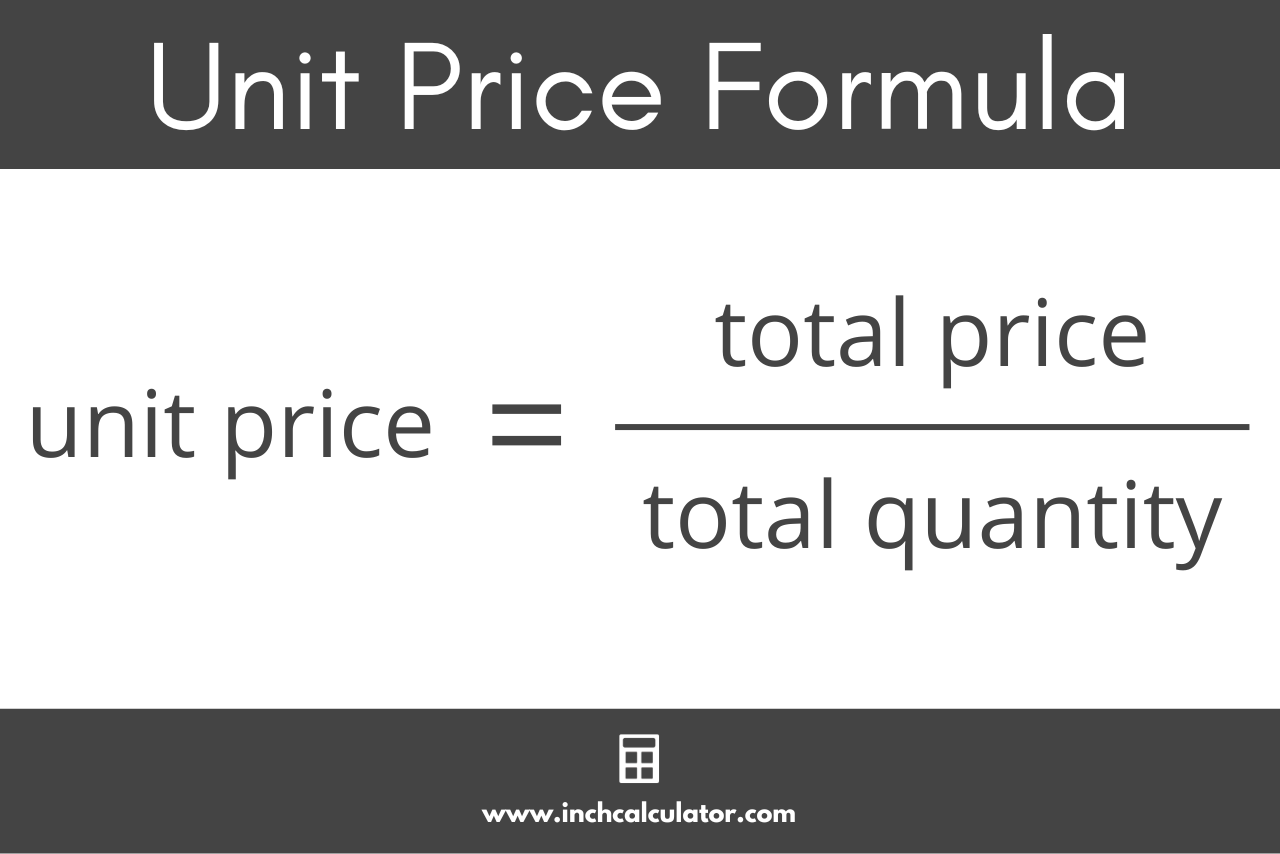# Unit Price Calculator

Enter the quantity and total price to calculate the cost per unit, or unit price.

Item One
\$
Item One
\$
Item One
\$
Item One
\$
Item One
\$
Item One
\$

## Unit Price:

Item One
\$
per item
Learn how we calculated this below

## How to Calculate Unit Price

The unit price can be found using a simple formula if the quantity and total cost are known. Simply divide the total price by the total quantity to find the unit price.

The formula for calculating unit price is:

unit price = total price ÷ total quantity

Thus, the unit price is equal to the total price divided by the total quantity.## How to Calculate Unit Price for Different Units of Measure

In some cases, the quantity may be measured in a different unit than the unit price; for instance, if the total quantity is measured in pounds but the unit price is measured in ounces.

To calculate the unit price, first convert the total quantity to the desired unit. Thus, if the quantity is measured in ounces but you want to find the unit price in pounds, convert the quantity to pounds first, then calculate the unit price using the formula above.

Our unit conversion utilities make this simple.

For example, let’s find the unit price per pound for a quantity of 32 ounces at a price of \$6.38.

Start by converting 32 ounces to pounds.

32 oz ÷ 16 oz/lb = 2 lbs

Next, calculate the unit price using the formula above.

unit price = \$6.38 ÷ 2 lbs
unit price = \$3.19 per lb

Thus, the unit price is \$3.19 per pound in this example.

Overall, it’s important to understand how to calculate the unit price of items you are purchasing so that you know how much they will cost.